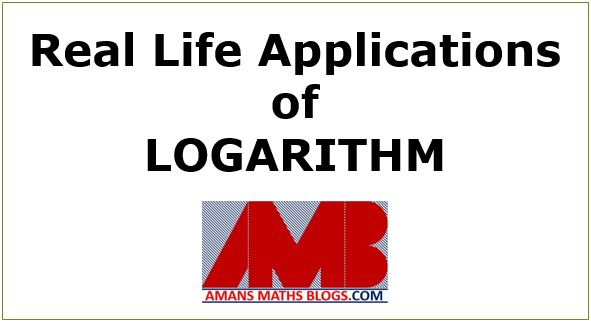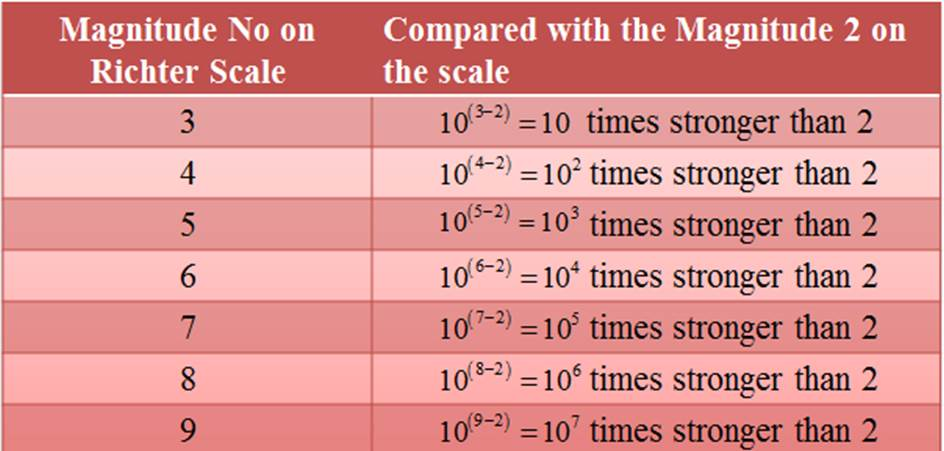Saturday, June 12, 2021
Home > Latest Announcement > Real Life Scenario of Logarithm

# Real Life Scenario of LogarithmDownloading Link is given at Last

# Real Life Application of Logarithms

Real life scenario of logarithms is one of the most crucial concepts in our life. As we know, in our maths book of 9th-10th class, there is a chapter named LOGARITHM is a very interesting chapter and its questions are some types that are required techniques to solve. Therefore, you must read this article “Real Life Application of Logarithms” carefully.

Apart from Real Life Application of Logarithms, you can also clear you following doubts:

But, after solving these questions and getting knowledge of logarithm, Have you think that why you study this chapter what is the use of LOGARITHM in our daily life. The LOGARITHM is used in the following application of real life.real life scenario of logarithms

## Real-Life Application of Logarithm Earthquake Intensity Measurement

To know about the real-life scenario of logarithms we start as an earthquake intensity measurement. For this, first we let to know some knowledge related to the earthquake measurement instrument known as Seismograph. which produces a graph output known as Seismograph.real life scenario of logarithms

In the earthquake, a Seismic wave produces which travels through the Earth layer. The seismic wave gives out an energy that causes the earth to shake and also gives out low frequency acoustic energy.

Read More : 129 Maths Short Tricks
Now, these seismic waves is recorded by the seismograph instrument and its output is the seismographs graph. It is a record of the ground motion in three co-ordinate axes (x, y, and z), with the z-axis perpendicular to the Earth’s surface and the x- and y- axes parallel to the Earth’s surface.

An earthquake’s hypo-center is the position where the strain energy stored in the rock is first released, marking the point where the fault begins to rupture. This occurs directly beneath the epicenter, at a distance known as the focal depth.

The amplitude of the seismic waves decreases with distance. Now, the instrument seismograph is based on a logarithmic scale, which is developed by Charles Richter in 1932 devised the first magnitude scale for measuring earthquake magnitude.

This is commonly known as the Richter scale. The magnitude of an earthquake is calculated by comparing the maximum amplitude of the signal with this reference event at a specific distance.## Real life scenario of logarithms

The Richter scale is a base-10 logarithmic scale, which defines magnitude as the logarithm of the ratio of the amplitude of the seismic waves to an arbitrary, minor amplitude.where A is the amplitude of the earthquake recorded by the seismograph taken from 100 km (approx) from the epicenter of the earthquake and S is the standard earthquake whose amplitude is 1 micron approx. The magnitude of the standard earthquake isSince the Richter scale is a base-10 logarithmic scale, each number increase on the Richter scale indicates an intensity ten times stronger than the previous number on the scale.

For example: if we note the magnitude of the earthquake on the Richter scale as 2, then the other next magnitude on the scale is explained in the following table.Now according to the Richter scale magnitude of the earthquake, there is a lot of bad effect on our environments which may be a danger to the real world. Its details are given below in the table.

Richter MagnitudeDescriptionEarthquake Effects
0-2.0MicroNever Felt by People
2.0-2.9MinorFelt But Not Recorded
3.0-3.9MinorFelt But Not Damaged Cost
4.0-4.9LightCeiling Lights Swing But Not Damaged
5.0-5.9ModerateAffects weak construction and cause wall crack
6.0-6.9StrongAffects area up to 160 km from the epicenter
7.0-7.9MajorAffect area up to further area and cause several damaged
8.0-8.9GreatAffect area beyond 100 miles and cause severe damaged
9.0-9.9GreatAffect area beyond 1000 miles with disastrous effects
10+EpicNever Been Recorded

This is one of the real-life scenario of logarithms, which must be known.

## Real Life Application of Logarithms in Determining pH Value

The Real-Life scenario of Logarithms is to measure the acidic, basic or neutral of a substance that describes a chemical property in terms of pH value. To know this concept in detail, click here.

## Real-Life Application of Logarithms in Measuring Sound Intensity

As we knew that the sound carries energy and it is defined as I = P/A, where P is the power through which the energy E flows through per unit area A which is perpendicular to the direction of travel of the sound wave.

Now, according to physics rule, the sound intensity is measured in terms of loudness which is measured in terms of a logarithm. Thus the sound intensity is defined as the. In this definition, dB is the decibels. It is one-tenth of bel(B) and I and I0 are the sound intensity.

If I = I0, then.

If I = 10I0, thenetc. Hence, we can different values.## Real Life Application of Logarithm in Calculating Complex Values

Sometimes we need to find the values of some complex calculations like x = (31)^(1/5) (5th root of 31), finding a number of digits in the values of (12)^256 etc. To solve these types of problems, we need to use the logarithms.

The solving method of these problems will be learning in another maths blogs post. The URL of the post will be mentioned below in the future.

Practice: Logarithm Questions Set 1

error: Content is protected !!